M403K 2nd Midterm Exam Solutions
Exam Given March 7, 2002

Problem 1. Graphing

Consider the function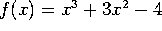. (This function factors as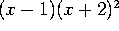).

a) Find the partition points of f (if there are any) and make a sign chart for f.

Since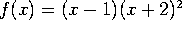, the partition points are at x=-2 and x=1. Evaluating test points f(-3)=-58 < 0, f(0)=-4 < 0, and f(2)=16 > 0 shows that f(x) is NEGATIVE for x < -2, NEGATIVE for -2 < x < 1, and POSITIVE for x > 1.

b) Find the critical points of f (if there are any) and make a sign chart for f'.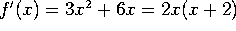, so our critical points are at x=-2 and x=0. Test each region: f'(-3)=9 > 0, f'(-1)=-3 < 0, f'(1)=9 > 0, so f'(x) is POSITIVE for x < -2, NEGATIVE for -2 < x < 0, and POSITIVE for x > 0.

c) Find the inflection points of f (if there are any) and make a sign chart for f''.

f''(x) = 6x+6, so our only inflection point is at x=-1. Testing f''(0) and f''(2) shows that f''(x) is NEGATIVE for x < -1 and POSITIVE for x > -1.

d) Sketch the graph of the function. Show clearly the location of the partition points, critical points and inflection points, and the value of the function at these special points. Be sure that the positivity, direction, and curvature of your graph agree with your calculations in parts a-c.

[I can't sketch on screen, but here's a description]. Up until x=-2, f(x) is negative, increasing, and curving down. The graph reaches a local maximum at (-2,0) - -2 is both a partition point and a critical point. Between -2 and -1, the function is negative, decreasing, and curving down. (-1, -2) is an inflection point. Between x=-1 and x=0, the function is negative, decreasing, but curving up, reaching a local minimum at (0,-4). Between 0 and 1, the function is negative, increasing, and curving up, hitting the x-axis at (1,0). Past 1 it is positive, increasing, and curving up.

Problem 2. Max-min

Find the absolute maximum and absolute minimum values of the function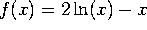on the interval [1,3].

First compute f'(x) = (2/x)-1. This is zero at x=2, which is our only critical point. (If you want, you can use the second derivative test to see that this is a local maximum, but that's not necessary). The extrema must be among our endpoints and critical points. Comparing values, we see that f(1)=-1,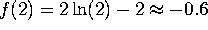, and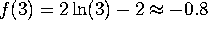. The maximum value of -0.6 is achieved at x=2 and the minimum value of -1 is achieved at x=1.

Problem 3. Wild West WIMPs

Widget Industries of Missoula and Pocatello (WIMP) is offering high-tech digital widgets to their customers in Montana and Idaho. They estimate that the demand x is related to the price p by the demand equation x=100-p. Their cost function is C(x)=40x+600.

a) Write down the revenue R(x) and the profit P(x) as functions of x.

p=100-x, so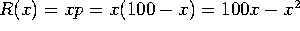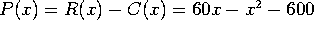.

b) Find the value of x that maximizes revenue. At that production level, what will the price be? How much revenue will the company bring in?

The maximum revenue is when R'(x)=0. Since R'(x) = 100-2x, this occcurs at x=50. The price is then 50, and the revenue is 2500.

c) Find the value of x that maximizes profit? How big is that maximum profit?

The maximum profit is when P'(x)=0. Since P'(x) = 60-2x, this occcurs at x=30. The maximum profit is P(30)=300.

Problem 4. Implicit differentiation

Consider the curve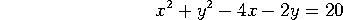.

a) Find dy/dx at the point (x,y).

2 x dx + 2 y dy - 4 dx - 2 dy = 0, or (2x-4)dx + (2y-2)dy =0. Solving for dy/dx gives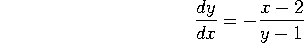b) Find the slope of the line tangent to the curve at the point (5,5).

At x=5, y=5, dy/dx = -(5-2)/(5-1) = -3/4.

c) Estimate the value of y when x=5.04.

dy = (dy/dx)dx = (-3.4)(0.04) = -0.03, so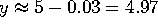.

Problem 5. Exponential Growth

A piece of cheese left in the refrigerator too long has become a biology experiment. The mold on it is growing exponentially, with a growth rate of 10%/day (compounded continuously!). Currently there are 3 grams of mold.

a) How much mold will there be in x days? (Write down a formula, with all the numbers except x filled in).

Initial value =3, growth rate = 0.1, time =x, so quantity of mold is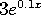b) How long will it take for the mold to double?

Doubling time is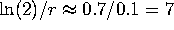days.

c) When will there be 24 grams of mold on the cheese? Simplify your answer as much as possible.

You need to double 3 times, so it takes 21 days. Alternatively, solve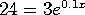, hence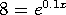, hence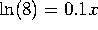, so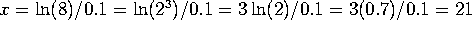days.

Problem 6. Exponentials and logs

For a, b, and c, take the derivatives, with respect to x, of the following functions. Your final answer should be expressed entirely in terms of x. (e.g.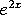, not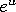). However, in these parts you do NOT need to simplify your results further.

a)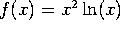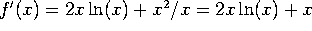.

b)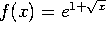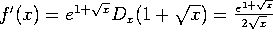c)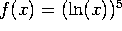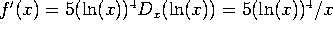d) Simplify the expression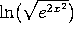.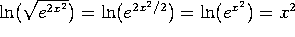.